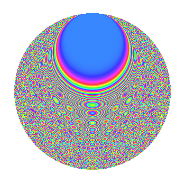# Properties

 Label 731.2.uLevel 731 Weight 2 Character orbit u Rep. character $$\chi_{731}(52,\cdot)$$ Character field $$\Q(\zeta_{21})$$ Dimension 696 Newforms 2 Sturm bound 132 Trace bound 2

# Related objects

## Defining parameters

 Level: $$N$$ = $$731 = 17 \cdot 43$$ Weight: $$k$$ = $$2$$ Character orbit: $$[\chi]$$ = 731.u (of order $$21$$ and degree $$12$$) Character conductor: $$\operatorname{cond}(\chi)$$ = $$43$$ Character field: $$\Q(\zeta_{21})$$ Newforms: $$2$$ Sturm bound: $$132$$ Trace bound: $$2$$ Distinguishing $$T_p$$: $$2$$

## Dimensions

The following table gives the dimensions of various subspaces of $$M_{2}(731, [\chi])$$.

Total New Old
Modular forms 816 696 120
Cusp forms 768 696 72
Eisenstein series 48 0 48

## Trace form

 $$696q + 4q^{2} + 2q^{3} - 108q^{4} + 4q^{5} - 4q^{6} - 22q^{7} - 28q^{8} + 24q^{9} + O(q^{10})$$ $$696q + 4q^{2} + 2q^{3} - 108q^{4} + 4q^{5} - 4q^{6} - 22q^{7} - 28q^{8} + 24q^{9} - 8q^{10} - 16q^{11} - 4q^{12} - 18q^{13} - 22q^{14} - 16q^{15} - 60q^{16} - 24q^{18} - 4q^{19} + 40q^{20} + 52q^{21} + 32q^{22} - 28q^{23} + 16q^{24} + 32q^{25} - 20q^{26} - 16q^{27} + 10q^{28} - 40q^{29} - 76q^{30} - 104q^{31} + 10q^{32} + 34q^{33} + 4q^{34} + 44q^{35} - 290q^{36} - 14q^{37} - 58q^{38} - 62q^{39} + 204q^{40} + 36q^{41} - 66q^{43} - 196q^{44} - 40q^{45} + 4q^{46} + 44q^{47} + 202q^{48} - 314q^{49} + 58q^{50} - 16q^{51} - 22q^{52} + 20q^{53} - 24q^{54} - 100q^{55} + 114q^{56} - 112q^{57} - 56q^{58} - 46q^{59} - 164q^{60} + 22q^{61} - 86q^{62} - 34q^{63} - 224q^{64} - 136q^{65} - 52q^{66} - 50q^{67} - 22q^{69} - 152q^{70} + 32q^{71} + 102q^{72} + 56q^{73} + 154q^{74} - 86q^{75} - 56q^{76} + 154q^{77} + 96q^{78} + 14q^{79} + 20q^{80} - 194q^{81} - 64q^{82} + 106q^{83} + 440q^{84} + 52q^{85} + 144q^{86} - 156q^{87} + 280q^{88} - 70q^{89} + 386q^{90} + 150q^{91} + 24q^{92} + 40q^{94} - 194q^{95} - 156q^{96} + 98q^{97} - 84q^{98} + 34q^{99} + O(q^{100})$$

## Decomposition of $$S_{2}^{\mathrm{new}}(731, [\chi])$$ into irreducible Hecke orbits

Label Dim. $$A$$ Field CM Traces $q$-expansion
$$a_2$$ $$a_3$$ $$a_5$$ $$a_7$$
731.2.u.a $$348$$ $$5.837$$ None $$-2$$ $$5$$ $$3$$ $$-71$$
731.2.u.b $$348$$ $$5.837$$ None $$6$$ $$-3$$ $$1$$ $$49$$

## Decomposition of $$S_{2}^{\mathrm{old}}(731, [\chi])$$ into lower level spaces

$$S_{2}^{\mathrm{old}}(731, [\chi]) \cong$$ $$S_{2}^{\mathrm{new}}(43, [\chi])$$$$^{\oplus 2}$$